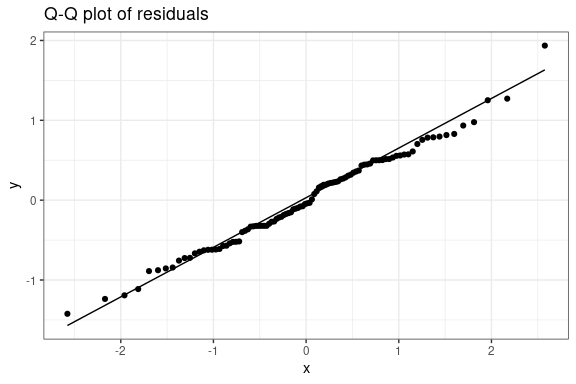# Using the tidytreatment package with BART

#### 2022-02-19

This vignette demonstrates an example workflow for heterogeneous treatment effect models using the BART package for fitting Bayesian Additive Regression Trees and tidytreatment for investigating the output of such models. The tidytreatment package can also be used with bartMachine models, support for bcf is coming soon (see branch bcf-hold on github).

## Simulate data

Below we load packages and simulate data using the scheme described by Hill and Su (2013) with the additional of 1 categorical variable. It it implemented in the function simulate_hill_su_data():


library(BART)
library(tidytreatment)
library(dplyr)
library(tidybayes)
library(ggplot2)

# set seed so vignette is reproducible
set.seed(101)

# simulate data
sim <- simulate_su_hill_data(n = 100, treatment_linear = FALSE,  omega = 0, add_categorical = TRUE,
coef_categorical_treatment = c(0,0,1),
coef_categorical_nontreatment = c(-1,0,-1)
)


Now we can take a look at some data summaries.


# non-treated vs treated counts:
table(sim$data$z)
#>
#>  0  1
#> 61 39

dat <- sim$data # a selection of data dat %>% select(y, z, c1, x1:x3) %>% head() #> y z c1 x1 x2 x3 #> 1 4.919484 1 2 -0.3260365 0.2680658 -0.1640324 #> 2 -1.342611 0 3 0.5524619 -0.5922083 -1.3832751 #> 3 -2.788457 0 3 -0.6749438 2.1334864 0.4235113 #> 4 2.089587 0 3 0.2143595 1.1727487 -0.7904889 #> 5 1.851633 0 1 0.3107692 0.7467610 1.2099248 #> 6 -5.903986 0 1 1.1739663 -0.2305087 0.8945168 ## Fit the regression model Run the model to be used to assess treatment effects. Here we will use BART, which is one implementation of Bayesian Additive Regression Trees in R (Chipman, George, and E. McCulloch 2010; Sparapani et al. 2016). The package can be found on CRAN. We are following the procedure in Hahn, Murray, and Carvalho (2020) (albeit without their more sophisticated model) where we estimate a propensity score for being assigned to the treatment regime, which improves estimation properties. The procedure is roughly as follows: 1. Fit ‘variable selection’ model (VS): Regress outcome against covariates (excluding treatment variable) 2. Select a subset of covariate from the VS model which are most associated with the outcome 3. Fit a ‘propensity score’ model (PS): A probit/logit model estimating the propensity score using only the covariates selected in step 2 4. Fit the treatment effect model (TE): Using the original covariates and propensity score from step 3  # STEP 1 VS Model: Regress y ~ covariates var_select_bart <- wbart(x.train = select(dat,-y,-z), y.train = pull(dat, y), sparse = TRUE, nskip = 2000, ndpost = 5000) # STEP 2: Variable selection # select most important vars from y ~ covariates model # very simple selection mechanism. Should use cross-validation in practice covar_ranking <- covariate_importance(var_select_bart) var_select <- covar_ranking %>% filter(avg_inclusion >= quantile(avg_inclusion, 0.5)) %>% pull(variable) # change categorical variables to just one variable var_select <- unique(gsub("c1[1-3]$","c1", var_select))

var_select

# STEP 3 PS Model: Regress z ~ selected covariates
# BART::pbart is for probit regression
prop_bart <- pbart(
x.train = select(dat, all_of(var_select)),
y.train = pull(dat, z),
nskip = 2000,
ndpost = 5000
)

# store propensity score in data
dat$prop_score <- prop_bart$prob.train.mean

# Step 4 TE Model: Regress y ~ z + covariates + propensity score
te_model <- wbart(
x.train = select(dat,-y),
y.train = pull(dat, y),
nskip = 10000L,
ndpost = 200L, #*
keepevery = 100L #*
)

#* The posterior samples are kept small to manage size on CRAN

## Extract the posterior (tidy style)

Methods for extracting the posterior in a tidy format is included in the tidytreatment.


posterior_fitted <- fitted_draws(te_model, value = "fit", include_newdata = FALSE)
# include_newdata = FALSE, avoids returning the newdata with the fitted values
# as it is so large. newdata argument must be specified for this option in BART models.
# The .row variable makes sure we know which row in the newdata the fitted
# value came from (if we dont include the data in the result).

posterior_fitted
#> # A tibble: 20,000 × 5
#> # Groups:   .row 
#>     .row .chain .iteration .draw   fit
#>    <int>  <int>      <int> <int> <dbl>
#>  1     1     NA         NA     1  4.21
#>  2     2     NA         NA     1 -2.49
#>  3     3     NA         NA     1 -2.10
#>  4     4     NA         NA     1  3.33
#>  5     5     NA         NA     1  1.22
#>  6     6     NA         NA     1 -6.74
#>  7     7     NA         NA     1  3.66
#>  8     8     NA         NA     1 10.5
#>  9     9     NA         NA     1  9.37
#> 10    10     NA         NA     1  8.21
#> # … with 19,990 more rows

# Function to tidy predicted draws also, this adds random normal noise by default
posterior_pred <- predicted_draws(te_model, include_newdata = FALSE)

## Use some plotting functions from the tidybayes package

Since tidytreatment follows the tidybayes output specifications, functions from tidybayes should work.


treatment_var_and_c1 <-
dat %>%
select(z,c1) %>%
mutate(.row = 1:n(), z = as.factor(z))

posterior_fitted %>%
left_join(treatment_var_and_c1, by = ".row") %>%
ggplot() +
stat_halfeye(aes(x = z, y = fit)) +
facet_wrap(~c1, labeller = as_labeller( function(x) paste("c1 =",x) ) ) +
xlab("Treatment (z)") + ylab("Posterior predicted value") +
theme_bw() + ggtitle("Effect of treatment with 'c1' on posterior fitted values")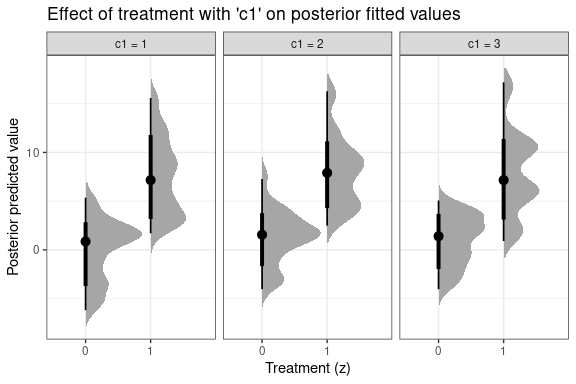## Calculate Treatment Effects

Posterior conditional (average) treatment effects can be calculated using the treatment_effects function. This function finds the posterior values of $\tau(x) = \text{E}(y ~ \vert~ T = 1, X = x) - \text{E}(y ~ \vert~ T = 0, X = x)$ for each unit of measurement, $$i$$, (e.g. subject) in the data sample.

Some histogram summaries are presented below.


# sample based (using data from fit) conditional treatment effects, posterior draws
posterior_treat_eff <-
treatment_effects(te_model, treatment = "z", newdata = dat) 

# Histogram of treatment effect (all draws)
posterior_treat_eff %>%
ggplot() +
geom_histogram(aes(x = cte), binwidth = 0.1, colour = "white") +
theme_bw() + ggtitle("Histogram of treatment effect (all draws)")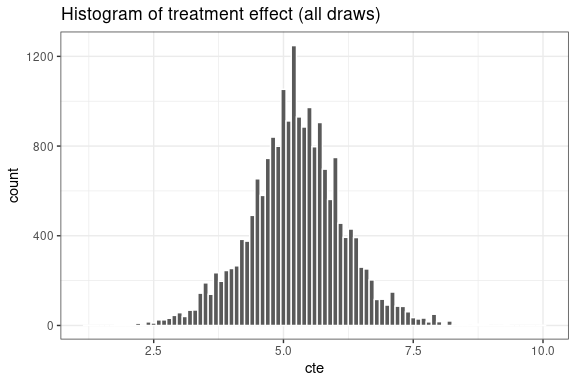# Histogram of treatment effect (median for each subject)
posterior_treat_eff %>% summarise(cte_hat = median(cte)) %>%
ggplot() +
geom_histogram(aes(x = cte_hat), binwidth = 0.1, colour = "white") +
theme_bw() + ggtitle("Histogram of treatment effect (median for each subject)")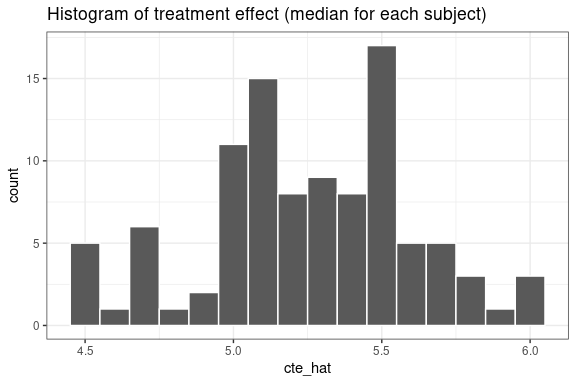# get the ATE and ATT directly:

posterior_ate <- tidy_ate(te_model, treatment = "z", newdata = dat)
posterior_att <- tidy_att(te_model, treatment = "z", newdata = dat)

We can create a trace plot for the treatment effect summaries easily too:


posterior_ate %>% ggplot(aes(x = .draw, y = ate)) +
geom_line() +
theme_bw() +
ggtitle("Trace plot of ATE")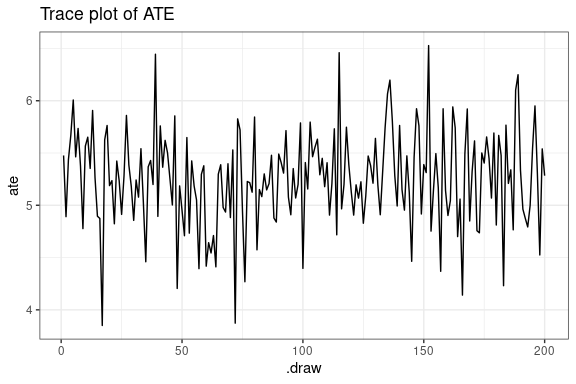We can also focus on the treatment effects for just those that are treated.


# sample based (using data from fit) conditional treatment effects, posterior draws
posterior_treat_eff_on_treated <-
treatment_effects(te_model, treatment = "z", newdata = dat, subset = "treated") 

posterior_treat_eff_on_treated %>%
ggplot() +
geom_histogram(aes(x = cte), binwidth = 0.1, colour = "white") +
theme_bw() + ggtitle("Histogram of treatment effect (all draws from treated subjects)")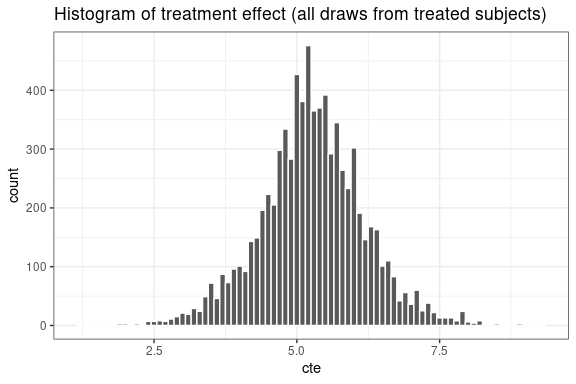Plots can be made that stack each subjects posterior CIs of the CATEs.


posterior_treat_eff %>% select(-z) %>% point_interval() %>%
arrange(cte) %>% mutate(.orow = 1:n()) %>%
ggplot() +
geom_interval(aes(x = .orow, y= cte, ymin = .lower, ymax = .upper)) +
geom_point(aes(x = .orow, y = cte), shape = "circle open", alpha = 0.5) +
ylab("Median posterior CATE for each subject (95% CI)") +
theme_bw() + coord_flip() + scale_colour_brewer() +
theme(axis.title.y = element_blank(),
axis.text.y = element_blank(),
axis.ticks.y = element_blank(),
legend.position = "none")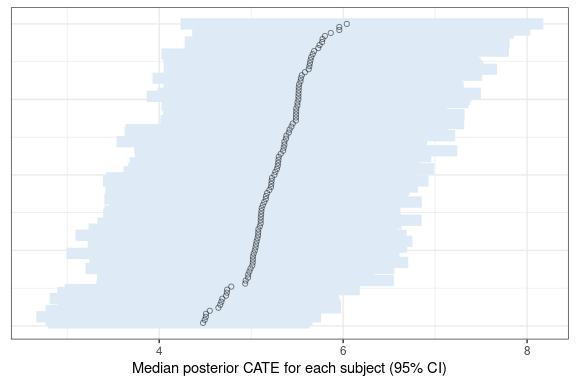We can also plot the CATEs varying over particular covariates. In this example, instead of grouping by subject, we group by the variable of interest, and calculate the posterior summaries over this variable.


posterior_treat_eff %>%
left_join(tibble(c1 = dat$c1, .row = 1:length(dat$c1) ), by = ".row") %>%
group_by(c1) %>%
ggplot() +
stat_halfeye(aes(x = c1, y = cte), alpha = 0.7) +
scale_fill_brewer() +
theme_bw() + ggtitle("Treatment effect by c1")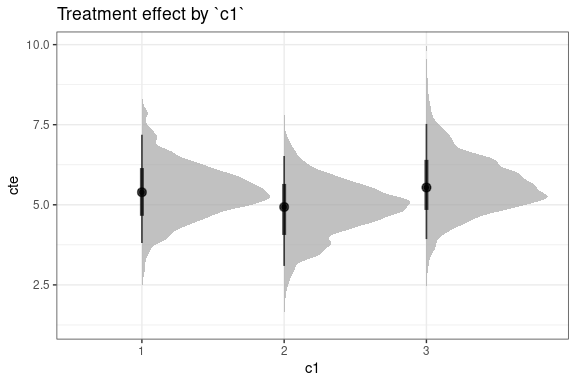## Common support calculations

Common support testing (Hill and Su 2013) can be tested directly, or a Boolean can be included when calculating the treatment effects.


# calculate common support directly
# argument 'modeldata' must be specified for BART models
csupp_chisq <- has_common_support(te_model, treatment = "z", modeldata = dat,
method = "chisq", cutoff = 0.05)

csupp_chisq %>% filter(!common_support)

csupp_sd <- has_common_support(te_model, treatment = "z", modeldata = dat,
method = "sd", cutoff = 1)
csupp_sd %>% filter(!common_support)

# calculate treatment effects (on those who were treated)
# and include only those estimates with common support
posterior_treat_eff_on_treated <-
treatment_effects(te_model, treatment = "z", subset = "treated", newdata = dat,
common_support_method = "sd", cutoff = 1)
#> Note: Argument 'newdata' must be original dataset when calculating common support.

## Investigating variable importance

We can count how many times a variable was included in the BART (on average) in conjunction with the treatment effect, or overall. This method uses a simple average of occurrences, see Bleich et al. (2014) for more sophisticated methods.


treatment_interactions <-
covariate_with_treatment_importance(te_model, treatment = "z")

treatment_interactions %>%
ggplot() +
geom_bar(aes(x = variable, y = avg_inclusion), stat = "identity") +
theme_bw() + ggtitle("Important variables interacting with treatment ('z')") + ylab("Inclusion counts") +
theme(axis.text.x = element_text(angle = 45, hjust=1))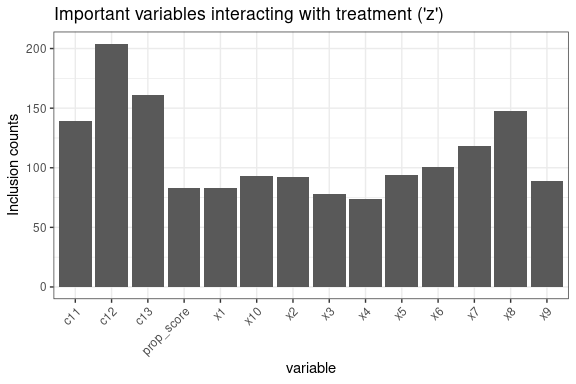variable_importance <-
covariate_importance(te_model)

variable_importance %>%
ggplot() +
geom_bar(aes(x = variable, y = avg_inclusion), stat = "identity") +
theme_bw() + ggtitle("Important variables overall") +
ylab("Inclusion counts") +
theme(axis.text.x = element_text(angle = 45, hjust=1))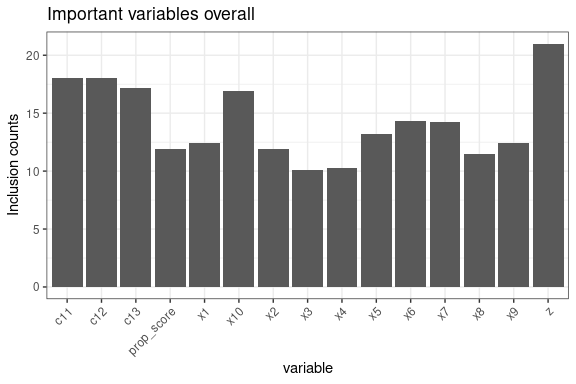## Model checking and convergence

Here are some examples of model checking we can do.

Code for trace plot of model variance ($$\sigma^2$$).


# includes skipped MCMC samples
variance_draws(te_model, value = "siqsq") %>%
filter(.draw > 10000) %>%
ggplot(aes(x = .draw, y = siqsq)) +
geom_line() +
theme_bw() +
ggtitle("Trace plot of model variance post warm-up")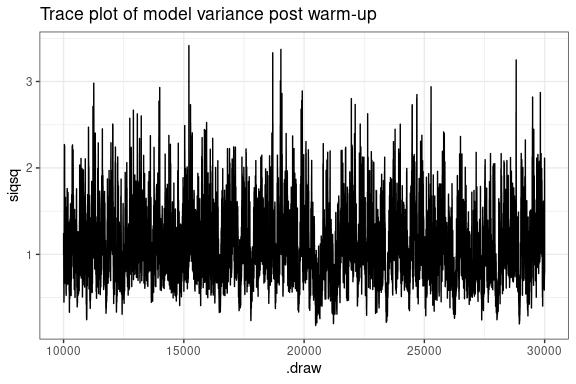Code for examining model residuals.


res <- residual_draws(te_model, response = pull(dat, y), include_newdata = FALSE)
res %>%
point_interval(.residual, y, .width = c(0.95) ) %>%
select(-y.lower, -y.upper) %>%
ggplot() +
geom_pointinterval(aes(x = y, y = .residual, ymin = .residual.lower,  ymax = .residual.upper), alpha = 0.2) +
scale_fill_brewer() +
theme_bw() + ggtitle("Residuals vs observations")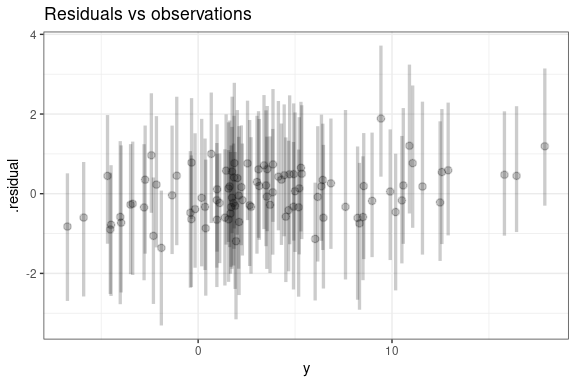res %>% summarise(.fitted = mean(.fitted), y = first(y)) %>%
ggplot(aes(x = y, y = .fitted)) +
geom_point() +
geom_smooth(method = "lm") +
theme_bw() + ggtitle("Observations vs fitted")
#> geom_smooth() using formula 'y ~ x'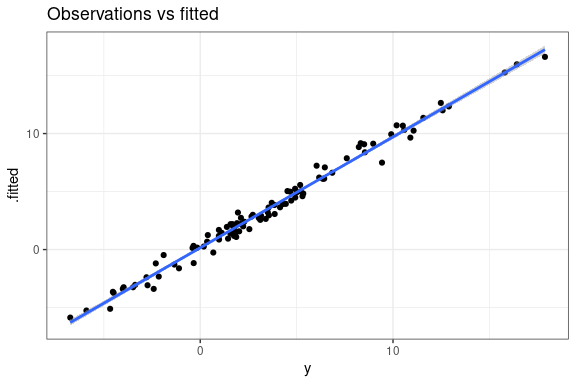res %>% summarise(.residual = mean(.residual)) %>%
ggplot(aes(sample = .residual)) +
geom_qq() +
geom_qq_line() +
theme_bw() + ggtitle("Q-Q plot of residuals")